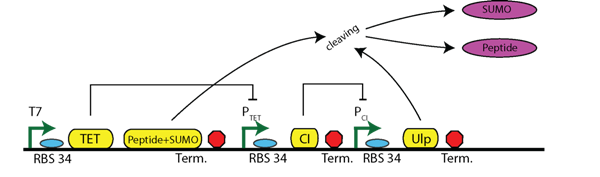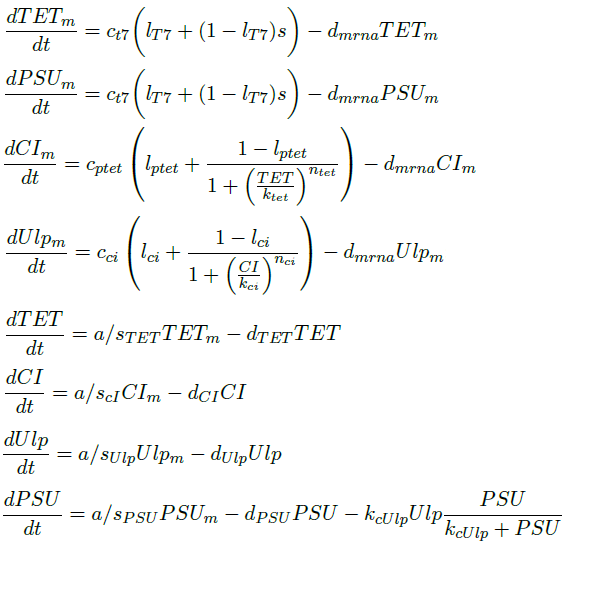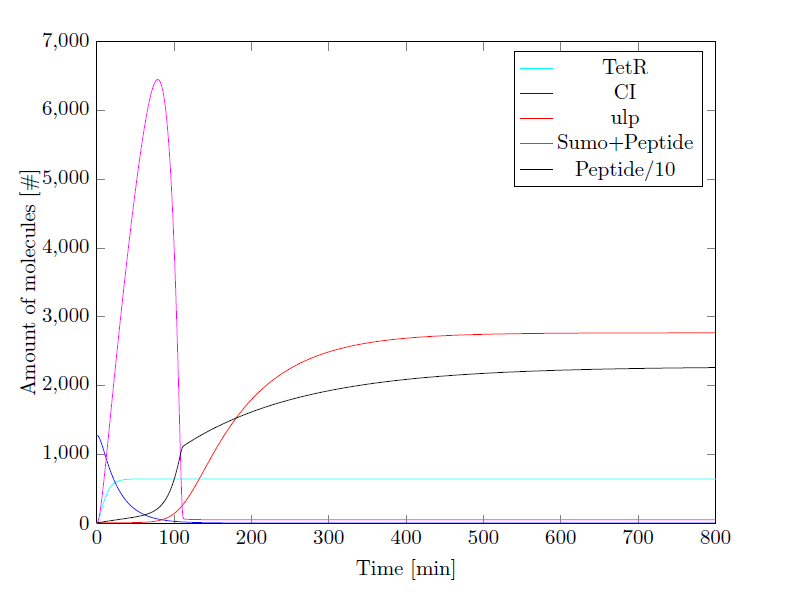# Timer Plus Sumo## Timer Plus Sumo

In this section the system of Figure 1 is modeled. The structure of the timer has two repressing promoters (PcI and Ptet) and the input is the T7 promoter and the output is the protease Ulp-1. This Ulp-1 cleaves off the SUMO from the produced SUMO-peptide.Figure 1: Circuit of the timer including sumo cleaving

## Differential Equations

The above circuit can be represented by the following differential equations. We assume a binary behavior of the T7 promoter. In the presence of IPTG, the T7 promoter will be active. So, we make the assumption that the T7 is binary variable with two possible states: either active 1 or inactive 0.## Parameters

 Parameter Value Description Units Reference ca 1020 Translation rate per amino acid min-1#a-1  cT7 4.16 Maximum transcription rate of T7 #m/min  cptet 2.79 Maximum transcription rate of Ptet #m/min  cci 1.79 Maximum transcription rate of Pci #m/min  dmRNA 0.231 Degradation rate of mRNA min-1  dTET 0.1386 Degradation rate of TET min-1  dCI 0.042 Degradation rate of CI min-1  dPEP 6.3*10-3 Degradation rate of the peptide min-1 Assumed the same as GFP dPSU 6.3*10-3 Degradation rate of the peptide plus SUMO min-1 Assumed the same as GFP dUlp 1.263*10-2 Degradation rate of Ulp min-1 Assumed the same as GFP lt7 0.002 Leakage factor of T7 - Assumption lptet 0.002 Leakage factor of Ptet - Assumption lci 0.002 Leakage factor of Pci - Assumption ktet 6 Dissociation constant of Ptet #m  kci 20 Dissociation constant of Pci #m  kcUlp 3 Turnover rate of Ulp min-1  nci 3 Hills coefficient -  ntet 3 Hills coefficient -  s 0 or 1 Activation/Inactivation of T7 promoter Binary Assumption sci 228 Length of CI amino acids  sPSU 18 + 110 Length of peptide plus SUMO amino acids  sTET 206 Length of TET amino acids  sUlp 233 Length of Ulp1 amino acids 

## Results

TET and ULP must be set equal to zero (or a numerical equivalent). For CI the steady state value is assumed as a starting condition as this is expressed before activation.Figure 2: Simulation Results